The LCAO Method

Introduction

In this report I shall describe a simple technique for estimating the ground-state energy of a many-atom system involves using the variational principle with a linear combination of single-atom wavefunctions. While sound in principle, a calculation using this method on the hydrogen molecule ion converges very slowly on the correct binding energy, for the reason that single-atom wavefunctions are not sufficiently dense in the region of overlap.

The Variational Principle

The variational principle is a simple and powerful method for establishing an upper bound on the ground-state energy of a quantum system. Given any wavefunction ψ, we may write ψ as a linear combination of eigenfunctions ψi of the Hamiltonian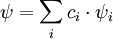(where, to meet the requirement of normalization, we require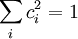Then, if we take the inner product of the wavefunction with the Hamiltonian H, we find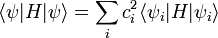Letting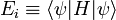be the energy associated with each eigenfunction, and letting ψ0 be the ground state, this becomes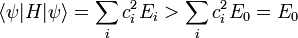or, more succinctly,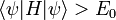If we have a large family of wavefunctions, we can calculate the expected energy for each one of them; with some amount of luck, one member of the family will be close to the actual ground-state wavefunction, and we'll have a good upper estimate for E0.

The LCAO Technique

The LCAO (Linear Combination of Atomic Orbitals) technique uses the variational principle together with a specific family of wavefunctions to find ground-state energies. Specifically, for many-atom systems, each electron will be influenced by a potential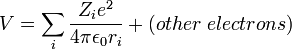where Zi refers to the number of protons in each atom and ri refers to the distance between the electron and each atom. Regardless of other electrons, if we consider locations very close to a particular atomic center (say, ri << 1 for a particular choice of i), we find that the non-relativistic Hamiltonian is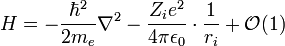To most significant order, then, this looks exactly like the Hamiltonian for the Hydrogen atom with a charge Ze instead of 1e at the center. As we come closer and closer, the agreement will become better and better as 1/ri dominates over the rest of the potential terms in the Hamiltonian. If ψznlm(r,θ,φ) are the wavefunctions for Hydrogen with z protons in the center nucleus, then we may well expect that a linear superposition of ground states, namely,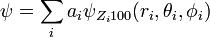(for some constants ai) will match the real ground-state wavefunction to most significant order.

What about higher-order corrections? While the linear combination of ground states is physically motivated, the LCAO technique for higher-order terms is more mathematically motivated. There will be some wave-function which is the ground state of the system; but any wave-function can be decomposed into sums of the eigenfunctions of the Hamiltonian for Hydrogen. Hence, no matter what the real ground-state wave-function looks like near the ith nucleus, we can decompose it into a sum of Hydrogen wave-functions. As this is true near any of the nuclei, we might expect that higher-order corrections to the potential will be reasonably matched by including higher-order wavefunctions of Hydrogen in our linear combination: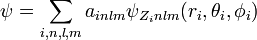But in general, in the limit of including the entire set of eigenfunctions, we will match the ground-state wavefunction. Hence, if the infinite vector space of functions in this family is denoted by Ψ, we find that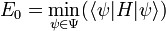A Computation Using LCAO

Background

How well does this work in practice, however? In order to find out, we consider the simplest possible non-Hydrogen system, namely singly ionized molecular Hydrogen. For this system, the Hamiltonian is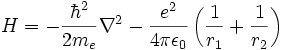where r1 and r2 are the distances from the electron to each proton. If we adopt natural units of length corresponding to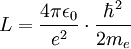(about 0.26 Angstrom) and natural units of energy corresponding to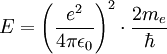(about 54.4 eV), the Hamiltonian reduces to the very simple form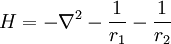If we place both protons on the z-axis, separated by a distance d, we can write the Hamiltonian in cylindrical coordinates, where it becomes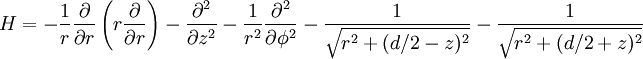Written in this way, it becomes explicit that the ground-state energy depends on the separation distance d. Hence, without knowing the value of d beforehand, we will have to calculate it as well: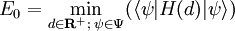To reduce the parameter space to a finite computational problem, we impose a cutoff on n, the highest order of the radial wave-functions of hydrogen which we consider. Additionally, we can exploit the symmetries of the Hamiltonian to note that the ground state must satisfy two conditions: ψ0(r,z,φ) = ψ0(r,z,0) (polar symmetry), and ψ0(r,z) = ψ0(r, - z) (mirror symmetry). Variation from these conditions implies a less-than-minimal value of -∇2, which in turn implies a larger value for the energy of the wavefunction.

These two conditions lead to the following restrictions on the coefficients ainlm: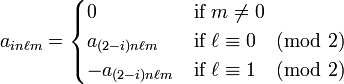The latter two come from the fact that the Legendre polynomials in the wavefunctions of Hydrogen are odd if l is odd, and they are even if l is even. Hence, while we expect the lowest-order guess for the wavefunction to be of the form

ψa = a1100100(r1,z1) + ψ100(r2,z2)]

The term involving the odd wavefunction ψ210 will be antisymmetric:

ψb = a1210210(r1,z1) - ψ210(r2,z2)]

Finally, one final computational aid may be realized. As the wavefunctions used are eigenfunctions of most of the Hamiltonian---except for the potential from the extra proton, we find that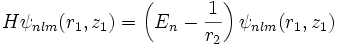and likewise,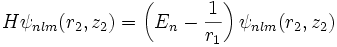where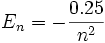is the energy of the nth radial state of the Hydrogen atom (in natural units).

With these considerations in effect, we are now ready to perform the variational calculation.

Computational Results

Starting with d = 4 and a1100 = a2100 = 1, we may perform a gradient descent in parameter space to find the lowest energy value of the system. The results of this process are shown in Table 1.

Maximal n d (Å) E0 (eV)
1 1.32 -1.76
2 1.27 -1.96
3 1.25 -2.07
4 1.24 -2.08
Table 1: Variational energies for the ionized hydrogen molecule. The code that generated these numbers is available here.

In Table 1, E0 refers to the binding energy of the system, namely, the energy of the electron and both protons relative to the energy of a free proton and a hydrogen atom. The column headed "Maximal n" refers to the largest n for which ainlm was taken to be nonzero.

While these values seem to be converging rapidly, this similitude is misleading. The experimentally-determined value of E0 is -2.65 eV , with a separation distance of 1.06 Å . This is not an effect of relativistic dynamics (as the electron energy is much less than 511 keV) and is not an effect of fine structure or other corrections (which would modify the energy at the hundredth of a percent level). The full energy of the electron predicted from the last entry in the table is -27.2 eV. Comparatively, the full energy from experiment would be -29.6 eV.

Why is the LCAO method so terrible in this case? A naive guess would be because we have stopped at n = 4. However, it is easy to see that adding further orbitals would result in mostly wasted efforts. The nth radial eigenfunction of Hydrogen depends on a characteristic radius na, where a = 2 in the natural units we have assumed. However, as the optimal distance d between the two protons is about 1.06 Å, or 4.0 in the natural units we have adopted, whereas the natural distance for the n = 5 wavefunctions is 10, it is easy to see why adding them will have little additional effect. Most of the variation in the n = 5 wavefunctions will happen at a radius beyond the distance between the two protons, whereas most of the interesting deviation in the ground-state wavefunction happens between the two protons. Hence, beyond n = 4 the Hydrogen wavefunctions represent a particularly feeble basis with which to describe the ground-state wavefunction of the Hydrogen ion molecule, and so converge very poorly to the correct result.

The moral of this report is that, for calculating energies (or wavefunctions) based on the variational principle, the basis functions of the wavefunction family under consideration must quickly converge to arbitrary functions in the region of overlap between any two independent potentials in the Hamiltonian, for otherwise the rate of convergence will be extremely slow.

© 2008 P. Behroozi. The author grants permission to copy, distribute and display this work in unaltered form, with attribution to the author, for noncommercial purposes only. All other rights, including commercial rights, are reserved to the author.

References

 J. Weiss, Nature, 165, 728 (1950).